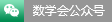Some recent domestic mathematical activities (Mar6 - Mar12, 2022)

Date：2022.03.6 - 2022.03.12

Introduction：Some recent domestic mathematical activities (Mar6 - Mar12, 2022). The information is taken from Tianyuan Mathematical Center in Northeast China.

Some recent domestic mathematical activities (Jan23 - Jan29, 2022)

Date：2022.01.23 - 2022.01.29

Introduction：Some recent domestic mathematical activities (Jan23 - Jan29, 2022). The information is taken from Tianyuan Mathematical Center in Northeast China.

Some recent domestic mathematical activities (Jan16 - Jan22, 2022)

Date：2022.01.16 - 2022.01.22

Introduction：Some recent domestic mathematical activities (Jan16 - Jan22, 2022). The information is taken from Tianyuan Mathematical Center in Northeast China.

Some recent domestic mathematical activities (Jan10 - Jan16, 2022)

Date：2022.01.10 - 2022.01.16

Introduction：Some recent domestic mathematical activities (Jan10 - Jan16, 2022). The information is taken from Tianyuan Mathematical Center in Northeast China.

Some recent domestic mathematical activities (Jan3 - Jan9, 2022)

Date：2022.01.03 - 2022.01.09

Introduction：Some recent domestic mathematical activities (Jan3 - Jan9, 2022). The information is taken from Tianyuan Mathematical Center in Northeast China.

Some recent domestic mathematical activities (Dec26, 2021 - Jan1, 2022)

Date：2021.12.26 - 2022.01.01

Introduction：Some recent domestic mathematical activities (Dec26, 2021 - Jan1, 2022). The information is taken from Tianyuan Mathematical Center in Northeast China.

Some recent domestic mathematical activities (Dec19 - Dec25, 2021)

Date：2021.12.19 - 2021.12.25

Introduction：Some recent domestic mathematical activities (Dec19 - Dec25, 2021). The information is taken from Tianyuan Mathematical Center in Northeast China.

Some recent domestic mathematical activities (Dec12 - Dec18, 2021)

Date：2021.12.12 - 2021.12.18

Introduction：Some recent domestic mathematical activities (Dec12 - Dec18, 2021). The information is taken from Tianyuan Mathematical Center in Northeast China.

Some recent domestic mathematical activities (Dec5 - Dec11, 2021)

Date：2021.12.05 - 2021.12.11

Introduction：Some recent domestic mathematical activities (Dec5 - Dec11, 2021). The information is taken from Tianyuan Mathematical Center in Northeast China.

Some recent domestic mathematical activities (Nov28 - Dec4, 2021)

Date：2021.11.28 - 2021.12.04

Introduction：Some recent domestic mathematical activities (Nov28 - Dec4, 2021). The information is taken from Tianyuan Mathematical Center in Northeast China.

The fourth BRICS Mathematics Conference

Date：2021.12.07 - 2021.12.10

Introduction：The fourth BRICS Mathematics Conference is going to be held from December 7-10, 2021 in a hybrid mode. The conference is co-founded by CMS. You are wecome to attend the conference virtually.

Heidelberg Laureate Forum，Heidelberg, Germany，Sep. 18–23, 2022

Date：2021.11.11 - 2022.02.11

Introduction：第9届 Heidelberg Laureate Forum (HLF)将于2022年9月18-22日在德国海德堡召开。欢迎青年学者（本科生、博士生和博士后）申请参会！申请日期：2021年11月11日至2022年2月11日。详见以下通知：

Some recent domestic mathematical activities (Nov21 - Nov27, 2021)

Date：2021.11.21 - 2021.11.27

Introduction：Some recent domestic mathematical activities (Nov21 - Nov27, 2021). The information is taken from Tianyuan Mathematical Center in Northeast China.

Some recent domestic mathematical activities (Nov14 - Nov20, 2021)

Date：2021.11.14 - 2021.11.20

Introduction：Some recent domestic mathematical activities (Nov14 - Nov20, 2021). The information is taken from Tianyuan Mathematical Center in Northeast China.

Some recent domestic mathematical activities (Nov7 - Nov13, 2021)

Date：2021.11.07 - 2021.11.13

Introduction：Some recent domestic mathematical activities (Nov7 - Nov13, 2021). The information is taken from Tianyuan Mathematical Center in Northeast China.

#### Professor Hua Loo-Keng is a famous mathematician

Professor Hua Loo-Keng is a famous Chinese mathematician and loves his motherland deeply. He devoted into the scientific career, and made an outstanding contribution to the Chinese mathematical career and talents cultivation for all his life.

#### Prof Chern is a worldwide math master

As a Chinese American mathematician, a foreign academician of Chinese Academy of Sciences, Professor Shiing Shen Chern is a worldwide math master. He made outstanding contributions to the development of mathematics.

#### Professor Jiaqing ZHONG

Professor Jiaqing ZHONG was very concerned about the development of the mathematics of the motherland during his lifetime and worked hard for it. He has said that the development of mathematics depends on the active cultivation and selection of outstanding young mathematicians. He sincerely wish to establish a fund in China to reward outstanding young mathematicians.#### WeChat

Scan two-dimensional code attention